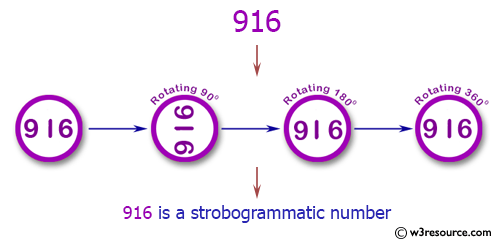# Strobogrammatic Numbers

### Definition

A number which is rotationally symmetrical, i.e., it'll appear the same when rotated by 180 deg in the plane of your screen. The following figure illustrates it better,(source: w3resource.com)

Given a number as the input, determine if it's strobogrammatic or not.

# Examples

• Truthy
1
8
0
69
96
69169
1001
666999
888888
101010101

• Falsey
2
3
4
5
7
666
969
1000
88881888
969696969


# Rules

• The number is guaranteed to be less than a billion.
• We are considering 1 in it's roman numeral format for the sake of the challenge.
• Input can be taken as number, or an array of chars, or as string.
• Output would be a truthy/falsey value. However, you are allowed to flip the output values.
• This is a , so fewest bytes will win!

• I think you should clarify which digits are rotationally symmetric with each other. The only way to figure it out right now is from the examples. Dec 23 '20 at 4:05

# Perl 5-pa, 48 34 bytes

@Arnauld had a better idea in his JavaScript answer than my first one in Perl, so I stole it.

y/0-9/01aaaa9a86/;$_="@F"==reverse  Try it online! # 05AB1E, 12 11 bytes •yΘOñ•I<èRQ  Try it online! -1 byte thanks to Razetime's suggestion Takes the number as a list of digits ## Explained •yΘOñ• # Push the number 1000090860 I< # Decrement the input list èR # And get each number when rotated 180 degrees, reversing it Q # Check if that equals the input  # Ruby-n, 30 bytes p$_.==\$_.reverse.tr'92-7','69'


Try it online!

• @Dingus yep, good catch. Dec 23 '20 at 8:38
• You may require just an extra -l flag to make it work for multiline inputs! ;-) Dec 23 '20 at 10:19

# Node.js 11.6.0,  34  33 bytes

Expects an array of digits. Returns false for strobogrammatic, or true otherwise.

n=>n.some(c=>n.pop()-c**66%79%63)


Try it online!

### How?

Taking the precision errors on large integers into account1, we look for a function:

$$f(x)=(x^p\bmod m_0)\bmod m_1$$

such that:

$$f(0)=0,\:f(1)=1,\:f(6)=9,\:f(8)=8,\:f(9)=6$$

and:

$$f(x)>9\text{ for }x\in\{2,3,4,5,7\}$$

1: These errors actually depend on the JS engine. This is guaranteed to work with Node 11.6.0.

# JavaScript (ES6), 38 bytes

Expects an array of digits. Returns false for strobogrammatic, or true otherwise.

n=>n.some(c=>n.pop()!="01----9-86"[c])


Try it online!

• The conditions say However, you are allowed to flip the output values. So it seems to me that ! can be removed and save one byte Dec 23 '20 at 5:51

# Python 3, 53 bytes

lambda n:["01....9.86"[int(c)]for c in n[::-1]]==[*n]


Try it online!